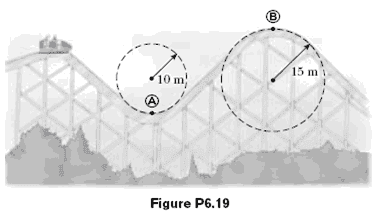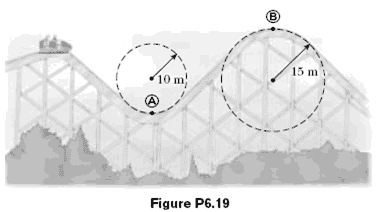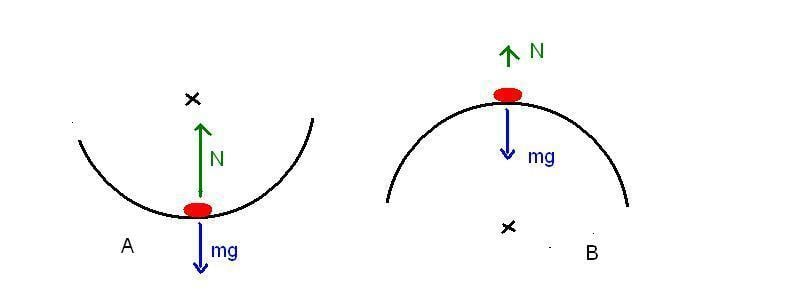# Centripetal force roller coaster problem

• Jahnic

## Homework Statement

A roller coaster car has a mass of 500 kg when fully loaded with passengers.(a)If the vehicle has a speed of 20.0 m/s at point A, what is the force exerted by the track on the car at this point?(b)What is the maximum speed the vehicle can have at B and still remain on the track?## Homework Equations

ac = v^2/r
F = m*a

My Question

My problem is not so much how to solve this, I'm rather concerned about centripetal forces in general.
For both questions a and b I intuitively know the result of an increased speed, however it doesn't make sense with my mechanical knowledge.
For question b, and this will probably solve my issue with question a, if the centripetal force is acting downwards why would an increasing speed and hence stronger downward force result in the train leaving the tracks. I suppose it either has to do something with Newtons first law or I'm misunderstanding something about centripetal forces.
Another related issue I have is radial acceleration. I know that radial acceleration = - centripetal acceleration but I don't understand the benefit and the implications of this notation.

Last edited:

## Homework Statement

A roller coaster car has a mass of 500 kg when fully loaded with passengers.(a)If the vehicle has a speed of 20.0 m/s at point A, what is the force exerted by the track on the car at this point?(b)What is the maximum speed the vehicle can have at B and still remain on the track?## Homework Equations

ac = v^2/r
F = m*a

My Question

My problem is not so much how to solve this, I'm rather concerned about centripetal forces in general.
For both questions a and b I intuitively know the result of an increased speed, however it doesn't make sense with my mechanical knowledge.
For question b, and this will probably solve my issue with question a, if the centripetal force is acting downwards why would an increasing speed and hence stronger downward force result in the train leaving the tracks. I suppose it either has to do something with Newtons first law or I'm misunderstanding something about centripetal forces.
Another related issue I have is radial acceleration. I know that radial acceleration = - centripetal acceleration but I don't understand the benefit and the implications of this notation.

What they call 'centripetal force' doesn't act downwards all of the time. At point b) it acting upwards. It is outward from the center of rotation to the position of the object. Is that your question?

What they call 'centripetal force' doesn't act downwards all of the time. At point b) it acting upwards. It is outward from the center of rotation to the position of the object. Is that your question?

It does, thank you. Question is now, why is it acting upward? I thought centripetal accelerations are always directed towards the center of the circular motion and hence also the centripetal force.

It does, thank you. Question is now, why is it acting upward? I thought centripetal accelerations are always directed towards the center of the circular motion and hence also the centripetal force.

Good point. I've confused 'centripetal' and 'centrifugal' again. They are equal and opposite and neither is a real 'force'. What's really happening is at the top of the track at point b) you need an 'centripetal' acceleration pointing downward to stay on the track. If gravity doesn't suffice to provide that acceleration then you will fly of the track. The only real force here is gravity. At point a) the track needs to support both the centripetal acceleration and the force of gravity so they add.

Good point. I've confused 'centripetal' and 'centrifugal' again. They are equal and opposite and neither is a real 'force'. What's really happening is at the top of the track at point b) you need an 'centripetal' acceleration pointing downward to stay on the track. If gravity doesn't suffice to provide that acceleration then you will fly of the track. The only real force here is gravity. At point a) the track needs to support both the centripetal acceleration and the force of gravity so they add.

Excuse my ongoing confusion but out of the two I thought only centrifugal forces are not real. If centripetal forces are not real why are we considering them for part a and why does the track need to support both gravitational and centripetal forces? At point A the centripetal force points upwards towards the center of the motion and in opposite direction of the gravitational force. Wouldn't that counteract weight, reduce the normal force and therefore the force exerted by the tracks on the car?
There's must be some kind of error in my thinking about centripetal forces here.

There are two "real" forces acting on the car: one is gravity, mg, downward. The other is the normal force from the ground, N. The resultant of the two has to give the centripetal force, which is needed to keep the car on track at the given speed. The centripetal force has to point towards the centre of the circular path. It is Fcp= mv2 /R=N-mg, upward in situation A. In case B, Fcp=mg-N, downward. The track can only push the car. If mg-mv2/R <0, gravity is no enough to provide the motion and the car will fly off the track.